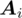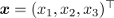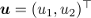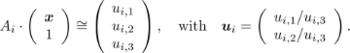# Left Heart Ventricle Data sets and Calibration

• We provide simulated dynamic and static projection images of a human heart with a contrasted left ventricle, aorta and myocardium.

All binary data is stored in row-major order little-endian format. This means, e.g. a projection(u,v) is stored by z images, where each image consists of v rows and each row consists of u columns.

# World Coordinate System and Projection Matrices

• In order to perform a 3-D reconstruction using the created projection data set we need to define the world-coordinate system of our object space and the basic relationship between a point in world coordinates and a projection image.

#### World Coordinate System

The origin of our 3-D world-coordinate system is set to the C-arm iso-center, i.e. the center of rotation. The space unit is set to millimeter.

#### Projection Matrices

The geometry of the image acquisition needs to be defined. The data sets are based on a real-world C-arm calibration. The problem with real-world systems is, that the C-arm is wobbling and does not move exactly on a circular trajectory. Therefore for each projection image a projection matrix in homogenous coordinates is calibrated. The projection matrix of the i-th projection image, in the following denoteddefines the perspective projection of a voxelin world coordinates and a pixelof the i-th projection image. It is given by:The projection matrix encodes a lot of system variables, e.g. the pixel size, rotation, etc.

Here the 3x4 projection matrices which encode the perspective projection of a voxel in the world-coordinate system onto the i-th projection image can be downloaded: